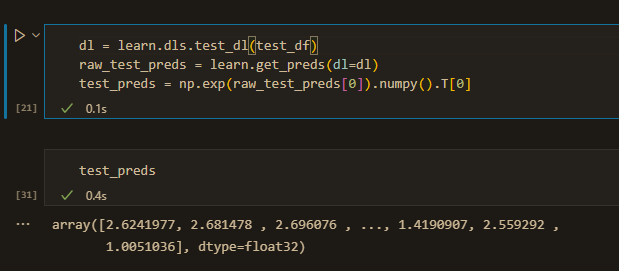# How to use predictions for a category block for tabular data?

Dear all,

`to = TabularPandas(df,y_names="Label",cont_names = cont_columns,splits=splits,y_block=CategoryBlock(),procs=[Normalize])`

My model is generated like this.

The dataframe has a “Label” column which is binary, 0 or 1.

I am trying to get predictions on a new dataframe like this:However, my predictions (the label) are not coming as 0’s or 1’s. What kind of a decoding should I do to get the correct prediction?

Hi,
I believe the preds are coming from your model. Maybe we can run an example on your model to figure out what’s going on.
Which model did you assign to your Learner?
BR,
Raviv

I am not sure what you mean by “which model did you assign to your learner”?

Hi,
When constructing the Learner we assign all kinds of parameters (Loss func, DLS, etc…) one of them is the Learner model:
learn = Learner(dls, model, loss_func=myLoss, metrics=myAccuracy)
I think we can find the problem if we will run the model and check his outputs
BR,
Raviv

Ah I see.

Here’s the code for my learner:

`learn = tabular_learner(dls, metrics=accuracy)`

I used the tutorial here: Tabular training | fastai

But I’m using a different dataset.